### Solving a difficult KenKen puzzle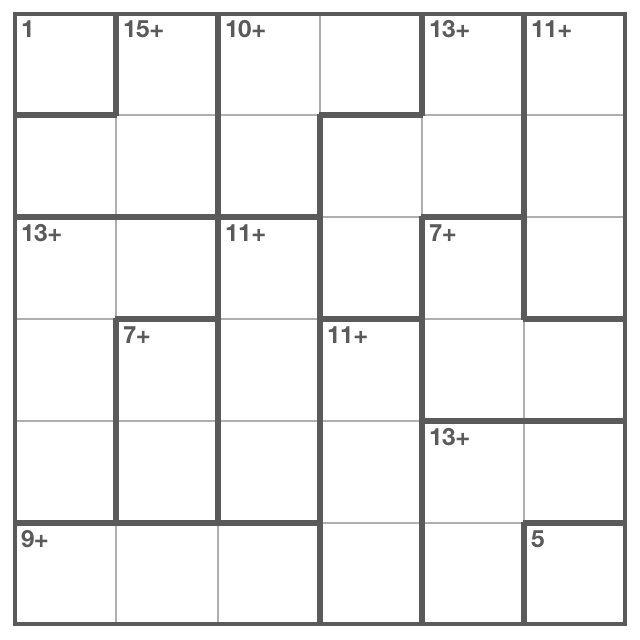That right there is the hardest KenKen puzzle I’ve ever come across. Maybe I’m just missing a strategy and there’s something obvious I overlooked, but I found the levels of ambiguity in this one to be very, very difficult to unravel. I’ve been coming back to this puzzle for months. Having finally cracked it, I wanted to document the solution. Partly to remember what happened, but mostly because it just felt so darn good to finally figure out.

With KenKen puzzles, there is no guessing. The puzzles are logical, and after doing hundreds of them over the past several years, I’ve only come across one or two that seemed to have equally forked possiibilities. And I hated those.

KenKen is similar to Sudoku, rows and columns contain n-digits with no repeats (a Latin Square). With KenKen, it can be helpful to know the sum of all digits in the puzzle’s dimension since every row and column must add up to that Triangular number. For a six digit puzzle this is 21. For this particular puzzle, it’s also worth remembering that there are only three paths to 11: `{1, 4, 6}`, `{2, 3, 6}` and `{2, 4, 5}`. Note the digits unique to each pathway.

### Starting Out

The key to breaking into this one was column 6. Since column 6’s top cage sums to 11, and F6 is 5, the sum of D6 and E6 must equal 5. Since the only possibilities are combinations of 1, 2, 3 and 4, and E6 can’t be 1 because the sum of E5 and F5 can’t be 12, there are only three possibilities, D6: `{1, 2, 3}` and E6: `{2, 3, 4}`. Since the sum of both cages is 20 and we know 5 is accounted for, the sum of C3 through F6 must be 15. That means A5 and B5 have to add up to 6. The remaining half of that cage, B4 and C4 must add up to 7.

We now know the sum of B4 through F6, so A4 must be 3. This also reveals F3 is also 3 because the sums of all cells in the top two cages of column 3 are known.

Having a 3 in F3 means the remaining slots of that cage, F1 and F2 can only be 2 and 4. This means F4 must be 1 and F5 must be 6 because, like E6, F5 can’t be 1. Additionally, D4 and E4 must contain 4 and 6 because there’s no other way to get to 11 using a 1. The last two cells of column 4 can only be 2 and 5.

The upper left cage, A2, B1 and B2, has limited choices. Since the largest possible number is 6, the sum of any two adjacent sides can’t be less than 9. Likewise, since the largest adjacent sum is 11, neither A2 or B1 can be less than 4. The only exception is B2 which could be 3 since both adjacent cells could be 6.

But the D2, E2 cage can’t contain 3 and 4. If it did, then A2 and B2 could only be 5 and 6, which would mean B1 would have to be 4. The problem is that F1 and F2 can only be 2 or 4 and column 2’s 4 would already be taken by the 3 and 4 in D2 and E2. So, the D2, E2 cage can only be a combination of 2 and 5 or 1 and 6, the sum of A2, B2 must be 9 and B1 has to be 6.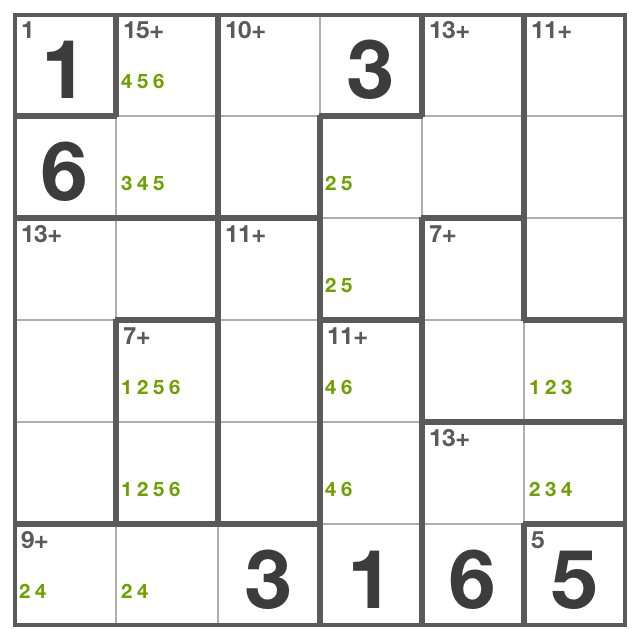At this point, things get hairy. I couldn’t see any obvious connection and essentially started trying alternate solutions.

There are only two remaining combinations for reaching 11 in the A6, B6, C6 cage, but if A2 was 6 and B2 was 3, the only remaining combination would be `{1, 4, 6}` because the top row would already have 6, 1, and 3, leaving only a 2 or 4 as candidates in A6 except the 2 would be taken by any combination of A3, B3, B4, A5 and B5. So, from here, the top row could only be filled out one way: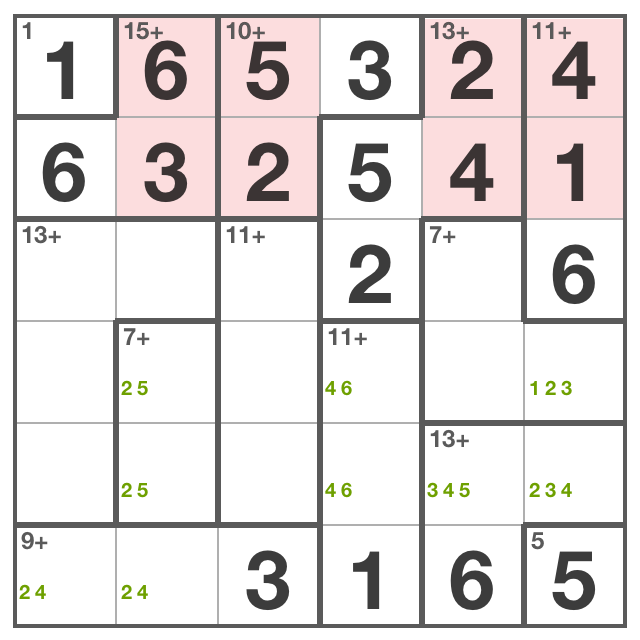That cascades down to E6. Now the D2, E2 cage has would have to be 2 or 5 because the 6 was taken by A2. But E6 couldn’t be 2 because then E5 would have to be 5 which would make E2 impossible. And here’s where this tangent falls apart all over the board. That was a long way to go to prove that A2 and B2 have to be 4 and 5.

### It all comes together

Disproving that was painful, but now we’re starting to get somewhere. Confirming A2‘s and B2‘s candidates reveals C2 is 3, F2 is 2 and F1 is 4. This also limits C1‘s candidates to 2 and 5. C4 is also limited to 2 and 5, so no other cells in row C can contain a 2 or 5.

Even better, there’s now only one way to fill in the top two rows: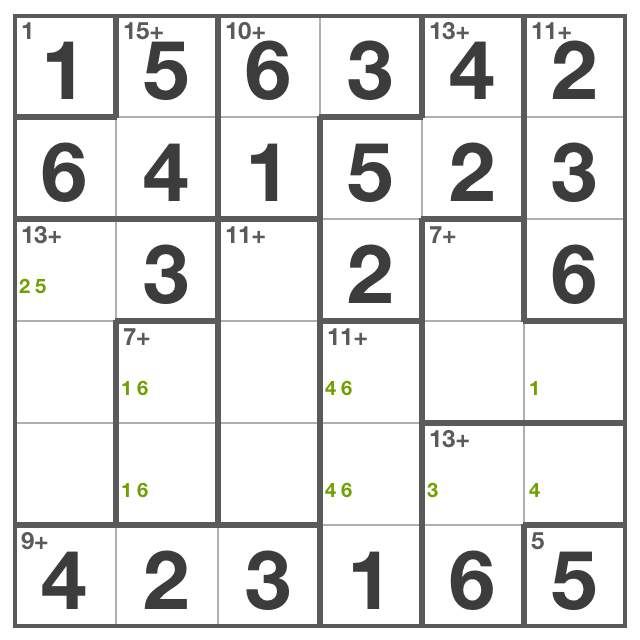That pretty much gives it all away. Columns 2, 4 and 6 are quickly revealed and the remaining values are easily discovered.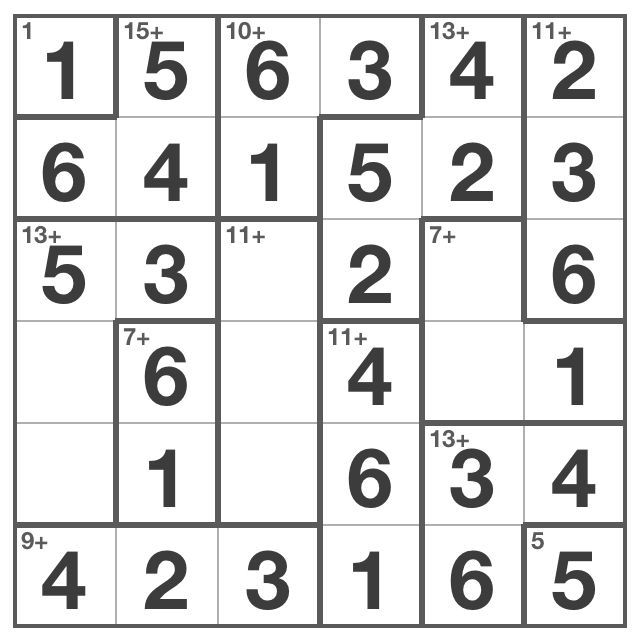The solved puzzle: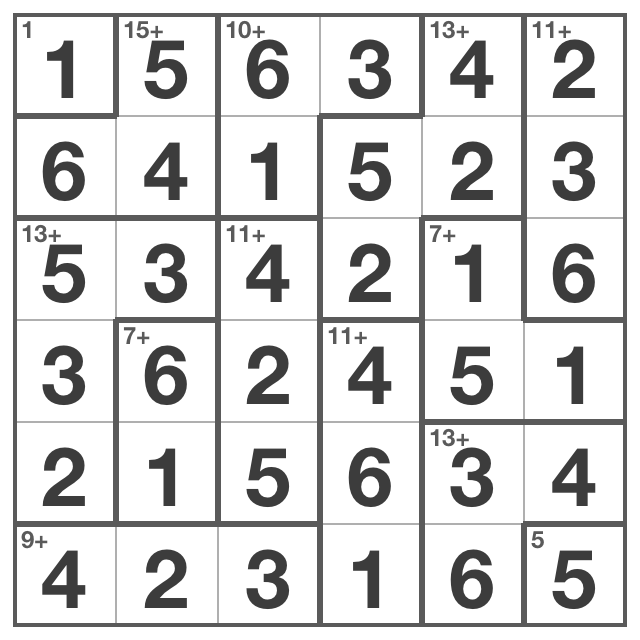### Solvers

It was interesting and humbling to run this puzzle through two KenKen solvers after finishing it by hand.

Michael Heyeck’s NekNek solver solved the puzzle instantly. Michael documented the creation of his KenKen solver, including the complete python source code (less than 150 lines with comments).

Below is the puzzle in JSON notation for the Poison Meatball solver. It was slightly reassuring that this took a few seconds to get to the answer.

``````{
"width": 6,
"height": 6,
"values": [1, 2, 3, 4, 5, 6],
"rules": [{
"op": "=",
"value": 1,
"cells": [{"x": 0, "y": 0 }]
}, {
"op": "+",
"value": 15,
"cells": [{"x": 1, "y": 0 }, {"x": 0, "y": 1 }, {"x": 1, "y": 1 }]
}, {
"op": "+",
"value": 10,
"cells": [{"x": 2, "y": 0 }, {"x": 3, "y": 0 }, {"x": 2, "y": 1 }]
}, {
"op": "+",
"value": 13,
"cells": [{"x": 4, "y": 0 }, {"x": 3, "y": 1 }, {"x": 4, "y": 1 }, {"x": 3, "y": 2 }]
}, {
"op": "+",
"value": 11,
"cells": [{"x": 5, "y": 0 }, {"x": 5, "y": 1 }, {"x": 5, "y": 2 }]
}, {
"op": "+",
"value": 13,
"cells": [{"x": 0, "y": 2 }, {"x": 1, "y": 2 }, {"x": 0, "y": 3 }, {"x": 0, "y": 4 }]
}, {
"op": "+",
"value": 11,
"cells": [{"x": 2, "y": 2 }, {"x": 2, "y": 3 }, {"x": 2, "y": 4 }]
}, {
"op": "+",
"value": 7,
"cells": [{"x": 4, "y": 2 }, {"x": 4, "y": 3 }, {"x": 5, "y": 3 }]
}, {
"op": "+",
"value": 7,
"cells": [{"x": 1, "y": 3 }, {"x": 1, "y": 4 }]
}, {
"op": "+",
"value": 11,
"cells": [{"x": 3, "y": 3 }, {"x": 3, "y": 4 }, {"x": 3, "y": 5 }]
}, {
"op": "+",
"value": 13,
"cells": [{"x": 4, "y": 4 }, {"x": 5, "y": 4 }, {"x": 4, "y": 5 }]
}, {
"op": "+",
"value": 9,
"cells": [{"x": 0, "y": 5}, {"x": 1, "y": 5}, {"x": 2, "y": 5}]
}, {
"op": "=",
"value": 5,
"cells": [{"x": 5, "y": 5}]
}]
}``````